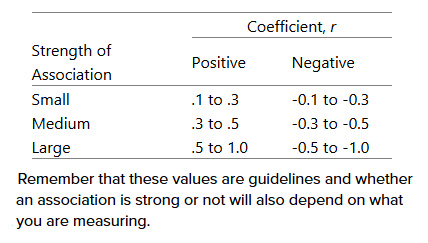# Statistical help needed for fMRI time-series

Hi everybody,

I am evaluating the effect of different multi-echo (ME) combination methods on the spatial maps and time-series of resting-state networks (after ICA). I am stuck with the statistical approach of determining whether the difference between the correlation of time-series of different combination methods is significant on a group level. As an example, I have the time-series from the Default Mode Network (DMN) from one subject after ME combination A and calculate the Pearson correlation with the time-series of the DMN of the same subject after ME combination B. This gives me a single correlation value and a single p-value. However, how do I calculate the group average statistics? So I would like to get a single p-value for the correlation of the DMN between combination method A and B for the whole group of subjects. I hope this is clear.

Could anybody help me?

Jesper

Hi Jesper,

Not a stats expert, but figured I can give it a shot anyway. Assuming that you are using the same subjects for the methods, you can do a paired t-test comparing the correlation values from method A to method B. This is also assuming the the correlation values are normally distributed. If they are not, you can Fisher/z-transform the values or do a non-parametric test (e.g. Wilcoxon signed-rank test). If you have more than two methods, then you will have to use something like an ANOVA test. Does this help (and if anyone has a better answer please speak up!)?

Steven

Hi Steven,

Thank you for your reply! The problem I face is that I only get one correlation value per method comparison (I only have one set of observations). For example, for subject 1 I compare/correlate the time-series resulting from method A and method B, resulting in a single correlation value and p-value. I think a paired t-test would have been applicable when I would have had a correlation value for method A and one for method B (two sets of observations) to test whether their distributions differ. Is this indeed what you thought my results looked like?

I am not a stats expert either so please correct me if I’m wrong!.